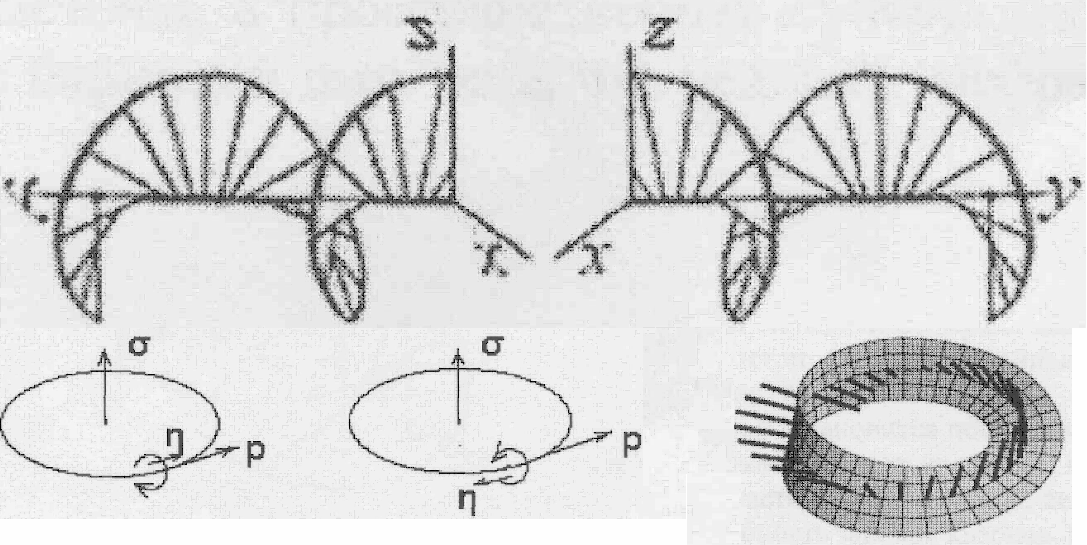# Theory EDCW (electrodynamics of curvilinear waves) of A.Kyriakos

Theory of EDCW (electrodynamics of curvilinear waves) of A.Kyriakos proceeds from a postulate that the physical vacuum exists and that it is filled with electromagnetic waves. The same postulate was used by Nikola Tesla in his researches. According to Kyriakos, elementary particles are formed as a result of a curvature of trajectories of electromagnetic waves (photons) and differ by quantity of coils and by direction of rotation of the currents arising in them (Fig. 1,2)Fig.1. Hypothesis of Kyriakos of generation of electron and positron from the wave of photon in the form of two simple cycles.Fig.2. Hypothesis of Kyriakos of generation of neutrino from the wave of photon in the form of double period cycle, laying on a Moebius band.

Movement of such curled photons submits to the equations of the Dirac's quantum field theory. Besides within the frameworks of the given hypothesis such known experimental facts can be explained as: dualism of wave - particle, division of all elementary particles on bozons and fermions, a simultaneous birth of electrons and positrons, absence of a mass of photon and occurrence of masses of elementary particles, spin and a charge of a particle, etc. Thus, many experimentally confirmed phenomena and effects of quantum electrodynamics can be described by bifurcations of electromagnetic waves. This is the main conclusion from the EDCW theory of A.Kyriakos.

However, EDCW theory does not give an answer to what are the electromagnetic waves and how they can be curled with generation of elementary particles. It is clear that such process should be described by some system of the equations, but not even the hint of what such system of the equations could be was presented in the EDCW theory. In addition EDSW theory lacking any connection with the theory of gravitation. Those are fundamental flaws of the theory.

At the same time, as it follows from the results of EDCW theory and Figs.1,2, the processes occurring in a microcosm can be successfully described by bifurcations in some nonlinear system of differential equations, and these are exactly those bifurcations (saddle - node and double period) which determine cascades of bifurcations of solutions complication in the universal Feigenbaum-Sharkovskii-Magnitskii (FSM) theory of transition to dynamical chaos in nonlinear systems of the macrocosm. Notably Moebius band plays the basic role in the theory FSM as the cycle of double period, being the first initial bifurcation of the original singular cycle which is located on its surface

Clearly, the basic system of the nonlinear equations cannot be the system of Maxwell's equations describing distribution of electromagnetic waves as, firstly, this system is linear, and secondly, it also isn’t associated in any way with the gravitation which is inherent to all material objects. Hence it appears that initial object of the uniform theory should be the object capable of generating elementary particles and which properties can be represented as electromagnetic and also gravitational fields. Basic nonlinear system of the equations should be the system of the equations describing dynamics of this initial object. It appears that such object with all necessary mathematical properties exists and it is the physical vacuum (ether). The ether is a dense compressible inviscid medium in three-dimensional Euclidean space that is described by density and perturbations velocity vector of the density.